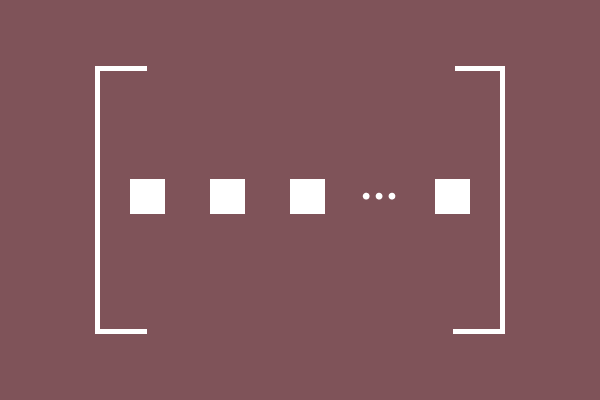Row Matrix

Definition

A matrix whose all elements are arranged in a row is called a Row matrix.Row matrix is one type of matrix and it is also called as a row vector. In this type of matrix, all elements are arranged in only one row but in different columns.

$M$ is a matrix in general form and it is a row matrix of order $1 \times n$.

$M = {\begin{bmatrix} e_{11} & e_{12} & e_{13} & \cdots & e_{1n}\\ \end{bmatrix}}_1 \times n}$

It can also be expressed in simple form.

$M = {\begin{bmatrix} e_ij} \end{bmatrix}}_m \times n}$

In this case of a Row vector , each element is displayed in one row. So, the row $i = 1$. Similarly, the number of rows $m = 1$. Therefore, a row matrix can be displayed in simple but in general form as follows.

$M = {\begin{bmatrix} e_1j} \end{bmatrix}}_1 \times n}$

Example

The following matrices are best examples for a row matrix.

$(1)\,\,\,\,$ $A = \begin{bmatrix} 5 \end{bmatrix}$

$A$ is a row matrix of order $1 \times 1$. Only one element is arranged in one row and one column in this matrix.

$(2)\,\,\,\,$ $B = \begin{bmatrix} -1 & 4 \end{bmatrix}$

$B$ is a row matrix of order $1 \times 2$. In this matrix, two elements are arranged in one row but in two columns.

$(3)\,\,\,\,$ $C = \begin{bmatrix} 9 & 4 & 3 \end{bmatrix}$

$C$ is a row matrix of order $1 \times 3$. Three elements are arranged in one row but in three columns in this matrix.

$(4)\,\,\,\,$ $D = \begin{bmatrix} 2 & 6 & 7 & 3 \end{bmatrix}$

$D$ is a row matrix of order $1 \times 4$. In this matrix, four elements are arranged in one row but in four columns.

All the row matrices are single row matrices but they have common shape and it is a rectangle. Hence, the row matrices are known as rectangular matrices.

Latest Math Topics
Latest Math Problems
Email subscription
Math Doubts is a best place to learn mathematics and from basics to advanced scientific level for students, teachers and researchers. Know more